# Importance factors from FORM method¶

Importance Factors are evaluated in the following context :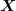denotes a random input vector, representing the sources of uncertainties,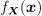its joint density probability,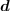a deterministic vector, representing the fixed variables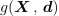the limit state function of the model,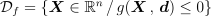the event considered here and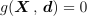its boundary (also called limit state surface).
The probability content of the event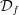is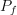:

(1)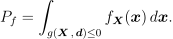In this context, the probabilitycan often be efficiently estimated by FORM or SORM approximations.
The FORM importance factors offer a way to rank the importance of the input components with respect the realization of the event. They are often interpreted also as indicators of the impact of modeling the input components as random variables rather than fixed values. The FORM importance factors are defined as follows.
The isoprobabilistic transformation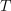used in the FORM and SORM approximation is a diffeomorphism from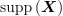into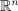, such that the distribution of the random vector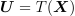has the following properties: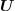and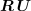have the same distribution for all rotations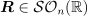.
In the standard space, the design point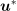is the point on the limit state boundary the nearest to the origin of the standard space. The design point is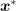in the physical space, where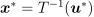. We note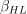the Hasofer-Lind reliability index: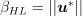.
When the-space is normal, the literature proposes to calculate the importance factor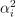of the variable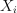as the square of the co-factors of the design point in the-space :

(2)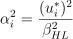This definition guarantees the relation :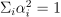.
Let’s note that this definition arises the following difficulties :
• Which signification for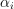when the variablesare correlated? In that case, the isoprobabilistic transformation does not associate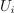tobutto a set of.

• In the case of dependence of the variables, the shape of the limit state function in the-space depends on the isoprobabilistic transformation and in particular on the order of the variableswithin the random vector. Thus, changing this order has an impact on the localization of the design point in the-space and, consequently, on the importance factors … (see [lebrun2009c] to compare the different isoprobabilistic transformations).

It is possible to give another definition to the importance factors which may be defined in the elliptical space of the iso-probabilistic transformation, where the marginal distributions are all elliptical, with cumulative distribution function noted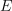, and not yet uncorrelated.The importance factorwrites:

(3)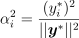This definition still guarantees the relation:.

Here, the event considered is established directly from the limit state function: this is the classical structural reliability formulation. However, if the event is a threshold exceedance, it is useful to explicit the variable of interest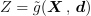, evaluated from the model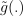. In that case, the event considered, associated to the threshold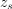has the formulation: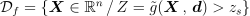and the limit state function is :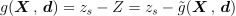.is the threshold exceedance probability, defined as :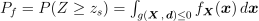. Thus, the FORM importance factors offer a way to rank the importance of the input components with respect to the threshold exceedance by the quantity of interest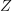. They can be seen as a specific sensitivity analysis technique dedicated to the quantity Z around a particular threshold rather than to its variance.